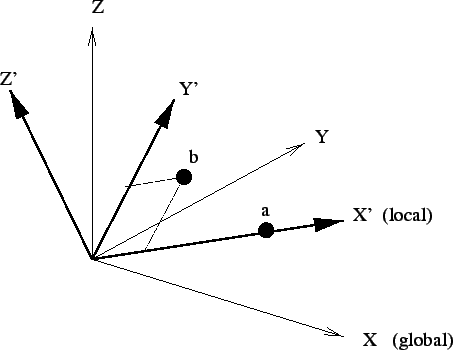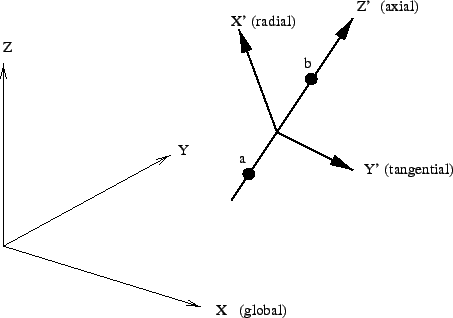Next: *OUTPUT Up: Input deck format Previous: *OBJECTIVE   Contents

## *ORIENTATION

Keyword type: model definition

This option may be used to specify a local axis system X'-Y'-Z' to be used for defining material properties. For now, rectangular and cylindrical systems can be defined, triggered by the parameter SYSTEM=RECTANGULAR (default) and SYSTEM=CYLINDRICAL.

A rectangular system is defined by specifying a point a on the local X' axis and a point b belonging to the X'-Y' plane but not on the X' axis. A right hand system is assumed (Figure 152).When using a cylindrical system two points a and b on the axis must be given. The X' axis is in radial direction, the Z' axis in axial direction from point a to point b, and Y' is in tangential direction such that X'-Y'-Z' is a right hand system (Figure 153).The parameter NAME, specifying a name for the orientation so that it can be used in an element property definition (e.g. *SOLID SECTION) is required (maximum 80 characters).

Notice that a shell ALWAYS induces a local element coordinate system, independent of whether an *ORIENTATION applies or not. For details the user is referred to Section 6.2.14.

For rectangular systems an additional rotation about one of the local axes can be specified on the second line underneath the *ORIENTATION card.

First line:

• *ORIENTATION
• Enter the required parameter NAME, and the optional parameter SYSTEM if needed.
Second line:
• X-coordinate of point a.
• Y-coordinate of point a.
• Z-coordinate of point a.
• X-coordinate of point b.
• Y-coordinate of point b.
• Z-coordinate of point b.
Third line (optional for local rectangular systems)
• local axis about which an additional rotation is to be performed
• angle of rotation in degrees.

Example:

*ORIENTATION,NAME=OR1,SYSTEM=CYLINDRICAL
0.,0.,0.,1.,0.,0.


defines a cylindrical coordinate system with name OR1 and axis through the points (0.,0.,0.) and (1.,0.,0.). Thus, the x-axis in the global coordinate system is the axial direction in the cylindrical system.

Example files: beampo2.Next: *OUTPUT Up: Input deck format Previous: *OBJECTIVE   Contents
guido dhondt 2018-12-15